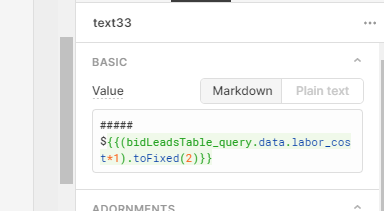# How do I make only 2 decimal places?

Use:

`(bidLeadsTable_query.data.profit_percent*100).toFixed(2).`

Anything with javascript it is worth googling something like "javascript 2 decimal places." You will get your answer pretty quick. I am a pretty experienced JS dev and I google stuff like this all the time!

1 Like

Thank you so much! I actually did, for about 45 minutes but I didn't know the syntax. I kept putting it outside the braces or in. I never thought to wrap it in () then call the function. Still fairly new but I'm getting there! Thanks again!

Though, I've noticed this only works when multiplying. So I'll be figuring that out next.It is so true that the more you know the better you are at googling stuff. Miss the right keyword and you are down the rabbit hole!

This should work for any math done inside of parentheses. If it doesn't check your formula.

1 Like

Agreed! But is there a solution that doesn't involve math? Where the "toFixed" function is applied to the object alone?

Ohh, you just want to set `bidLeadsTable_query.data.labor_cost.toFixed(2)` and that is not working?

`.toFixed()` only works on a number so probably `bidLeadsTable_query.data.labor_cost` is actually being typed as a string?

A couple of solutions. The first one you accidentally encountered and is the shortest, but not obvious.

`(bidLeadsTable_query.data.labor_cost *1).toFixed(2)` does a conversion to number. Never thought of doing it this way, but neat trick!

The other is to convert it to a number more deliberately and is probably the "right" way to do it:

`parseFloat(bidLeadsTable_query.data.labor_cost).toFixed(2)`

This confusion between number and string that looks like a number trips up someone every second of every day I am sure.

2 Likes

Haha, guess we both learned something new today but I'll start parsing just in case later on doing math gets in the way. Thanks again! Saved me a lot of headaches! lol.

It seems like Math.round() is a better solution, but it is not! In some cases it will NOT round correctly. Also, toFixed() will NOT round correctly in some cases.

To correct the rounding problem with the previous Math.round() and toFixed(), you can define a custom JavaScript round function that performs a "nearly equal" test to determine whether a fractional value is sufficiently close to a midpoint value to be subject to midpoint rounding. The following function return the value of the given number rounded to the nearest integer accurately.

``````Number.prototype.roundTo = function(decimal) {
return +(Math.round(this + "e+" + decimal)  + "e-" + decimal);
}

var num = 9.7654;
console.log( num.roundTo(2)); //output 9.77
``````